Learning Center > Using Sketchpad > Getting Started Tutorials > Angles in a Triangle

#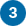Angles in a Triangle: Make and Prove a Conjecture

In this tutorial, you’ll explore properties of triangles by measuring the side lengths and angles of a triangle and using these measurements in calculations. By dragging the triangle and observing how the calculations change or stay the same, you’ll be able to make general conjectures that apply to all triangles. Then you’ll prove one of your conjectures.

### Sketchpad Skills | Introductory Movie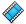In this and later tutorials, icons alone indicate when you must choose another tool. For example, before doing step 2 you must choose the Segment tool.

## Measure Lengths and AnglesMeasurements that you take with Sketchpad are dynamic—if you measure an angle or the length of a segment, the measurement will update automatically when you change the angle or the segment length. First you’ll measure the side lengths and angles of a triangle.

1.

Choose File | New Sketch to open a blank sketch.2.

Construct a triangle.3.

Label the vertices of the triangle A, B, and C.4.

Select the three sides of the triangle and choose Measure | Lengths.5.

Click, drag, and release to form a selection rectangle around point A (as pictured at right) so that the vertex and both sides of the angle—and nothing more—are selected.

6.

Choose Measure | Angle.

7.

Repeat to measure the other two angles. If you find you cannot measure an angle, it’s because you haven’t selected only one vertex and two sides. Click in empty space to deselect everything and try again.

8.

Drag the vertices and sides of your triangle and observe how the measurements change. What patterns do you notice?

## Use the CalculatorWhen using Sketchpad’s Calculator, you can click directly on a measurement to enter it into a calculation. Then, whenever the measurement changes, the calculation will automatically update as well.

9.

Choose Number | Calculate to open Sketchpad’s Calculator.

10.

Click a length measurement in the sketch to enter it into the Calculator. Then press the + key (on the Calculator or your keyboard), click another length measurement in the sketch, and click OK. The sum of the two lengths is displayed.11.

Drag the vertices of your triangle and observe how the sum of the lengths of the two sides compares to the length of the remaining side. What appears to be true for any triangle?

12.

Now focus on the angles. Use the Calculator to find the sum of the measures of all three angles of your triangle.13.

Drag the vertices of your triangle and observe the sum of the three angle measurements. What appears to always be true for any triangle?

## Communicate with Hot TextNow you’ll state a conjecture about the sum of a triangle’s angles and prove why your angle sum conjecture is true. You’ll use angle markers and a feature of Sketchpad called Hot Text to add mathematical notation to your caption.14.

Create an angle marker for each angle. To create an angle marker, click the vertex of an angle, drag into the angle, and release.

15.

Repeatedly click an angle marker to change the number of strokes (arcs) displayed. Give each of the three angle markers a different number of strokes. If you wish, you can also use Display | Color give each angle marker a different color.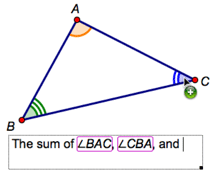16.

Click, drag, and release in empty space to form a caption rectangle.

17.

Write a mathematical statement using words and symbols to express your conjecture about the angle sum. Click angle markers to insert their names into the caption as Hot Text.18.

Roll over the angle names in your caption and notice how the angle markers light up in your sketch.To explain why your angle conjecture is true, you’ll construct a line through point A parallel to BC.

19.

Choose BC and point A and choose Construct | Parallel Line.20.

Add angle markers to the two new angles formed by the parallel line and the sides of your triangle.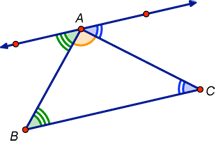21.

Select both new angle markers and choose Measure | Angles.

22.

Drag the vertices of your triangle. Which pairs of angles appear to always be congruent?

23.

Change the number of strokes so that congruent angles have the same number of strokes (and the same color if you used different colors).

24.

To prove your angle sum conjecture, you’ll start with a statement about the three angles that share a vertex at point A. How are these angles related?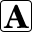25.

Create a caption. Use you observations in steps 22 and 24 to write a proof that justifies your conjecture about the sum of the angle measures in a triangle. Click objects in your sketch to insert them as Hot Text. Use the Text Palette for special symbols, such as (the congruent symbol).

## Explore More

26.

Construct a quadrilateral (or pentagon) and add angle markers. Find the sum of the measures of the angles and create a caption with Hot Text to present a conjecture.

27.

Prove your conjecture. (Hint: Start by constructing a diagonal of the polygon.) Use a caption with Hot Text to present your proof.